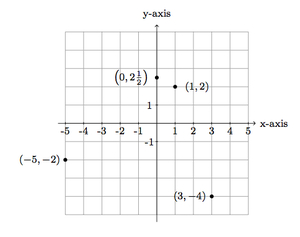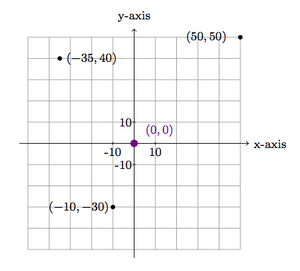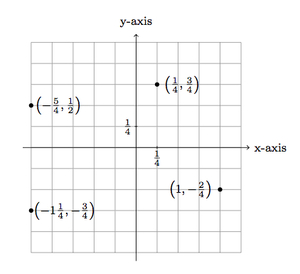# Plotting points in the coordinate plane

Alignments to Content Standards: 6.NS.C.6.c

1. For each set of points below, draw and label a set of coordinate axes and plot the points:

1. $(1,2), (3,-4), (-5,-2), \left(0,2\frac{1}{2}\right)$,
2. $(50,50), (0,0), (-10,-30), (-35,40)$,
3. $\left(\frac{1}{4},\frac{3}{4}\right)$, $\left(-\frac{5}{4},\frac{1}{2}\right)$, $\left(-1\frac{1}{4},-\frac{3}{4}\right)$, $\left(\frac{1}{4},-\frac{2}{4}\right)$.
2. How do the points influence your choice of scale for the axes?

## IM Commentary

The goal of this task is to provide experience labeling coordinate axes appropriately to plot a given set of points, which will mean choosing an appropriate scale. For this reason, the different sets of points have been chosen to encourage different scales. For the second set of points, for example, if students choose a scale of 1 (that is, each ''unit box'' on the coordinate axes is 1 by 1) it will be difficult to plot the points because the coordinate values are large. Similarly, for the third set of points, finding the fractional coordinates precisely works well if the units for the axes are fourths.

It is important to realize that these sets of points have been chosen so that there is a convenient scale for plotting all of them, which, of course, is not always possible. For example, plotting the points $(1,1), (1000,0), (0,1000000)$ will be difficult regardless of the scale. If the scale is small, it is not possible to show $(0,1000000)$. If the scale is large, then $(1,1)$ and $(1000,0)$ will look like the same point.

## Solution

1. The points are plotted and labeled below:Not all coordinates on the $y$-axis have been labeled as this would make the picture too crowded. Notice, too, that the the point $\left(0,2\frac{1}{2}\right)$ is halfway between $(0,2)$ and $(0,3)$.

2. The points are plotted and labeled below:This time only the first few squares are marked and each square is 10 units because the numbers that are being plotted are multiples of 10 (except for -35 which is halfway between -30 and -40). The point (0,0) has been highlighted as it is a special point in the coordinate system, the intersection of the two axes.

3. The points are plotted and labeled below:Each square here has been given a value of $\frac{1}{4}$ of a unit because the coordinates of our points are multiples of $\frac{1}{4}$. Notice that $1 = \frac{4}{4}$ and $\frac{1}{2} = \frac{2}{4}$.

1. When we choose a scale for the $x$ and $y$ axes, we want to choose a value which will make it as easy as possible to plot the points. For the first set of points, the coordinate values were mostly small whole numbers making it natural to mark off units of 1 on the axes. For the second set of points, the coordinates are mainly (small) multiples of 10, making it natural for each unit to count for 10 on the axes. Finally, in the third scenario, the coordinates are (small) multiples of $\frac{1}{4}$, making it natural for a unit to be worth $\frac{1}{4}$ in this case.